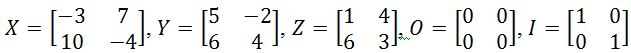# Properties of matrix multiplication

### Properties of matrix multiplication

In this section, we will learn about the properties of matrix to matrix multiplication. These properties include the associative property, distributive property, zero and identity matrix property, and the dimension property. You will notice that the commutative property fails for matrix to matrix multiplication. Lastly, you will also learn that multiplying a matrix with another matrix is not always defined. The product of the two matrices is only defined if the number of columns in the first matrix is equal to the number of rows in the second matrix.

#### Lessons

Let $X, Y, Z$ be matrices, $I_n$ be an identity matrix, and $O_n$ be a zero matrix. If all five of these matrices have equal dimensions, then we will have the following matrix to matrix multiplication properties:
Associative property
$(XY)Z=X(YZ)$

Distributive property
$X(Y+Z)=XY+XZ$
$(Y+Z)X=YX+ZX$

There are also some matrix to matrix multiplication properties with zero matrices and identity matrices.
Matrix to matrix multiplication property for the zero matrix
$OX=O$ or $XO=O$
Matrix to matrix multiplication property for the identity matrix
$XI_n=X$ or $I_n X=X$

Here are some important things to know.

Commutative property fails: Notice that the commutative property fails when you use matrix to matrix multiplication. For example, $XY \neq YX$.

Dimension property: When multiplying a matrix with another matrix, it is not always defined. The product of the two matrices is only defined if the number of columns in the first matrix is equal to the number of rows of the second matrix.
• Introduction
Properties of matrix to matrix multiplication overview:
a)
The basic matrix to matrix multiplication properties

b)
Failure of the Commutative property

c)
Dimension property

• 1.
Verifying the properties of matrix to matrix multiplication
You are given that. Verify that:
a)
$(XY)Z=X(YZ)$

b)
$X(Y+Z)=XY+XZ$

c)
$(Y+Z)X=YX+ZX$

d)
$OX=O$

e)
$IY=Y$

• 2.
Showing that the Commutative property fails
You are given that. Show that:
a)
$XY \neq YX$

b)
$X(Y+Z) \neq (Y+Z)X$

• 3.
Dimension Property
You are given that $X$ is a 2 x 4 matrix, $Y$ is a 3 x 3 matrix, and $Z$ is a 4 x 3 matrix. Are the following defined? If it is defined, show the dimensions of the matrix.
a)
$XY$

b)
$XZ$

c)
$ZX$

d)
$ZY$

e)
$XZY$

f)
$ZYX$